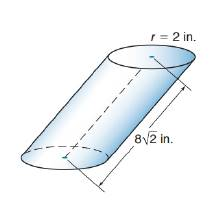Chapter 9.3, Problem 13EElementary Geometry For College St...

7th Edition
Alexander + 2 others
ISBN: 9781337614085

Solutions

Chapter
SectionElementary Geometry For College St...

7th Edition
Alexander + 2 others
ISBN: 9781337614085
Textbook Problem

Find the volume of the oblique circular cylinder. The axis meets the plane of the base to form a 45 ∘ angle.To determine

To calculate:

The volume of the oblique circular cylinder.

Explanation

Given:

The oblique circular cylinder has the indicated dimensions. The axis meets the plane of the base to form a 45 angle.

Formula used:

The volume of the right circular cylinder is V=πr2h.

Calculation:

Since the axis meets the plane of the base to form an 45 angle, the axis and the altitude form a right triangle.

From the figure,

sin45=h8212=h82

Still sussing out bartleby?

Check out a sample textbook solution.

See a sample solution

The Solution to Your Study Problems

Bartleby provides explanations to thousands of textbook problems written by our experts, many with advanced degrees!

Get Started

Simplify the expressions in Exercises 97106. (xy)1/3(yx)1/3

Finite Mathematics and Applied Calculus (MindTap Course List)

Find each limit. a. limx2| x2 |x2 b. limx2+| x2 |x2

Calculus: An Applied Approach (MindTap Course List)

Evaluate the limit. limx0e2xe2xln(x+1)

Single Variable Calculus: Early Transcendentals, Volume I